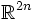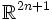# Difference between revisions of "Hard Whitney embedding theorem"

A compact connected differential manifold of dimension$n$ can be embedded inside$\R^{2n}$.
This is an improvement on the Whitney embedding theorem, which states that any compact connected differential manifold of dimension$n$ can be embedded inside$\R^{2n+1}$ and immersed inside$\R^{2n}$.